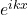## A particle of mass m is confined to a box of length. Its initial wave function is identical to that of the displacement of the string in th

Question

A particle of mass m is confined to a box of length. Its initial wave function is identical to that of the displacement of the string in the problem above, Boas Ch. 13, Sec. 4, #4.Find the solution of the Schrodinger equation

in progress 0
5 months 2021-09-04T17:28:07+00:00 1 Answers 6 views 0

φ = √2/L sin (kx),   E = (h² / 8 mL²) n²

Explanation:

The Schrödinger equation for a particle in a box is, described by a particle within a potential for simplicity with infinite barrier

V (x) =   ∞            x <0

0      0 <x <L

∞           x> L

This means that we have a box of length L

We write the equation

(- h’² /2m  d² / dx² + V) φ = E φ

h’= h / 2π

The region of interest is inside the box, since being the infinite potential there can be no solutions outside the box. The potential is zero

– h’² /2m d²φ/ dx² = E φ

The solution for this equation is a sine wave,

Because it is easier to work with exponentials, let’s use the reaction between the sine function and cook with the exponential= cos kx + i sin kx

Let’s make derivatives

dφ / dx = ika e^{ikx}

d²φ / dx² = (ik) e^{ikx} = – k² e^{ikx}

Let’s replace

– h’² / 2m (-k² e^{ikx}) = E e^{ikx}

E = h’² / 2m    k²

To have a solution this expression

Now let’s work on the wave function, as it is a second degree differential bond, two solutions must be taken

φ = A e^{ikx} + B e^{-ikx}

This is a wave that moves to the right and the other to the left.

Let’s impose border conditions

φ (0) = 0

φ (L) = 0

For being the infinite potential

With the first border condition

0 = A + B

A = -B

They are the second condition

0 = A e^{ikL}+ B e^{-ikL}

We replace

0 = A (e^{ikL} – e^{-ikL})

We multiply and divide by 2i, to use the relationship

sin kx = (e^{ikx} – e^{-ikx}) / i2

0 = A 2i sin kL

Therefore kL = nπ

k = nπ / L

The solution remains

φ = A sin (kx)

E = (h² / 8 mL²) n²

To find the constant A we must normalize the wave function

φ*φ = 1

A² ∫ sin² kx dx = 1

We change the variable

sin² kx = ½ (1 – cos 2kx)

A =√ 2 / L

The definitive function is

φ = √2/L sin (kx)